International
Tables for
Crystallography
Volume D
Physical properties of crystals
Edited by A. Authier

International Tables for Crystallography (2006). Vol. D, ch. 1.9, pp. 232-242

## Section 1.9.4. Graphical representation

W. F. Kuhsa*

aGZG Abt. Kristallographie, Goldschmidtstrasse 1, 37077 Göttingen, Germany
Correspondence e-mail: wkuhs1@gwdg.de

### 1.9.4. Graphical representation

| top | pdf |

Atomic displacement tensors (ADTs) described by their tensor coefficients may be represented graphically to clarify their physical meaning. Different graphical representations exist and will be discussed separately for second- and higher-order tensors in the following.

#### 1.9.4.1. Representation surfaces of second-order ADTs

| top | pdf |

Numerous examples of graphical representations of thermal-motion tensors (or, more generally speaking, atomic displacement tensors) have appeared in the literature since the early days of the computer program ORTEP written by C. K. Johnson (1965), yet the equal-probability surface usually displayed is only one of the possible representations of a second-order atomic displacement tensor. Representation surfaces are usually calculated in a Cartesian coordinate system. Accordingly, one has to transform the second-order ADT b into UC described in a Cartesian frame: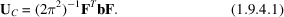The transformation matrix depends on the choice of Cartesian axes ei with respect to the reciprocal-cell axes ai (or equally well with respect to the direct axes ai). Choosing e1 along a1, e2 in the a1a2 plane and e3 completing the right-handed set, one obtains for the transformation matrix F (see also Willis & Pryor, 1975)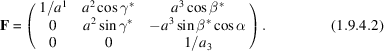Clearly, there is an infinite number of possible choices for relating a Cartesian frame to a crystallographic coordinate system with correspondingly different transformation matrices F (see e.g. Chapter 1.1of IT B). The most useful representation surface of the second-order atomic displacement tensor UC is the representation quadric defined by the tensor invariant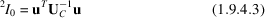where u is a displacement vector; U−1 is often called the variance–covariance matrix and has (in a general axes frame) covariant components. Under the conditions of positive definiteness,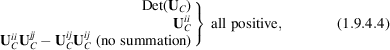the surface of the representation quadric is an ellipsoid whose semi-major axes (for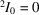) are of lengths equal to the root-mean-square displacements (r.m.s.d.'s) along the axes directions. The thermal vibration ellipsoids calculated in ORTEP are related to this surface; considering the discussion in Section 1.9.1, they should more appropriately be called atomic displacement ellipsoids or simply ORTEP ellipsoids. One notes that the Fourier transform of the atomic DWF, the atomic probability density function P(u), is given in the case of a second-order tensor as a trivariate Gaussian distribution,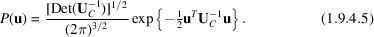On comparing (1.9.4.3)and (1.9.4.5), it is evident that (1.9.4.3)defines a surface of constant probability of finding a (displaced) atom. The integral of (1.9.4.5)over the volume inside the ellipsoid is a constant. For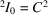with the integration limit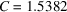(2.5003), the integral is equal to one half (nine tenths), and the ellipsoid is then called a 50 (90) per cent probability ellipsoid.

Other representation surfaces can be defined and are useful for special considerations. The quantities of interest are either the r.m.s.d.'s or the mean-square displacements (m.s.d.'s) defined in direct space. Here a distinction has to be made between the averaged squared displacement along a certain direction and the average for all squared displacements of an atom projected onto a given direction. Representation surfaces may also be calculated in reciprocal space, related to surfaces in direct space by Fourier transformation. For further details, see Nelmes (1969) and Hummel et al. (1990).

#### 1.9.4.2. Higher-order representations

| top | pdf |

Representation surfaces of higher-order tensors may be calculated from their invariants. While for second-order tensors surfaces can be found that fully describe the directional aspects of the tensor involved, higher-order tensors need several different surfaces for a full description (see e.g. Wondratschek, 1958; Sirotin, 1961). This makes the graphical representation of the displacements somewhat cumbersome and it is therefore rarely used. Instead, the probability density functions [given in equations (6.1.1.46), (6.1.1.48) or (6.1.1.49) of IT C] are calculated from the tensor coefficients and displayed in sections or as three-dimensional surfaces. If the higher-order terms are small, it is more appropriate to display only the difference between the total p.d.f. and the related Gaussian p.d.f., which may be calculated from the second-order displacement tensor using equation (1.9.4.5). Here, the second-order terms that were refined together with the higher-order terms are usually used (not the best-fitting second-order terms of a fit in the harmonic approximation):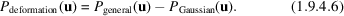The resulting anharmonic deformation densities (or disorder deformation densities in the case of static disorder) Pdeformation(u) may be displayed in a similar way to the total p.d.f.'s Pgeneral(u). The graphical representations appropriate for displaying those densities are similar to those used for electronic deformation densities (see e.g. Smith et al., 1977). A number of examples of displacement deformation densities of high symmetry are shown in Fig. 1.9.4.1as three-dimensional contour maps.Figure 1.9.4.1 | top | pdf |A selection of graphical representations of density modulations due to higher-order terms in the Gram–Charlier series expansion of a Gaussian atomic probability density function. All figures are drawn on a common scale and have a common orientation. All terms within any given order of expansion are numerically identical and refer to the same underlying isotropic second-order term; the higher-order terms of different order of expansion differ by one order of magnitude, but refer again to the same underlying isotropic second-order term. The orthonormal crystallographic axes are oriented as follows: x oblique out of the plane of the paper towards the observer, y in the plane of the paper and to the right, and z in the plane of the paper and upwards. All surfaces are scaled to 1% of the absolute value of the maximum modulation within each density distribution. Positive modulations (i.e. an increase of density) are shown in red, negative modulations are shown in blue. The source of illumination is located approximately on the  axis. The following graphs are shown (with typical point groups for specific cases given in parentheses). Third-order terms: (a) b222; (b) b223; (c) b113 = −b223 (point group); (d) b123 (point group). Fourth-order terms: (e) b2222; (f) b1111 = b2222; (g) b1111 = b2222 = b3333 (point group); (h) b1222; (i) b1112 = b1222; (j) b1122; (k) b1133 = b2233; (l) b1122 = b1133 = b2233 (point group). Fifth-order terms: (m) b22222; (n) b12223; (o) b11123 = b12223; (p) b11123 = b12223 = b12333 (point group). Sixth-order terms: (q) b222222; (r) b111111 = b222222; (s) b111111 = b222222 = b333333 (point group); (t) b112222; (u) b111133 = b222233; (v) b113333 = b223333; (w) b111122 = b112222 = b111133 = b113333 = b222233 = b223333 (point group); (x) b112233 (point group).

### References

Hummel, W., Raselli, A. & Bürgi, H.-B. (1990). Analysis of atomic displacement parameters and molecular motion in crystals. Acta Cryst. B46, 683–692.
Johnson, C. K. (1965). ORTEP – a FORTRAN thermal ellipsoid plot program. Report ORNL-3794. Oak Ridge National Laboratory, Tennessee, USA.
Nelmes, R. J. (1969). Representational surfaces for thermal motion. Acta Cryst. A25, 523–526.
Sirotin, Yu. I. (1961). Plotting tensors of a given symmetry. Sov. Phys. Crystallogr. 6, 263–271.
Smith, V. H. Jr, Price, P. F. & Absar, I. (1977). Representations of electron density and its topographical features. Isr. J. Chem. 16, 187–197.
Willis, B. T. M. & Pryor, A. W. (1975). Thermal vibrations in crystallography. Cambridge University Press.
Wondratschek, H. (1958). Über die Möglichkeit der Beschreibung kristallphysikalischer Eigenschaften durch Flächen. Z. Kristallogr. 110, 127–135.